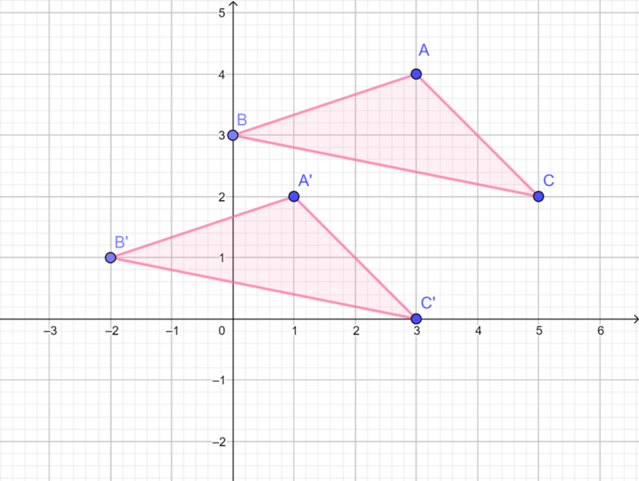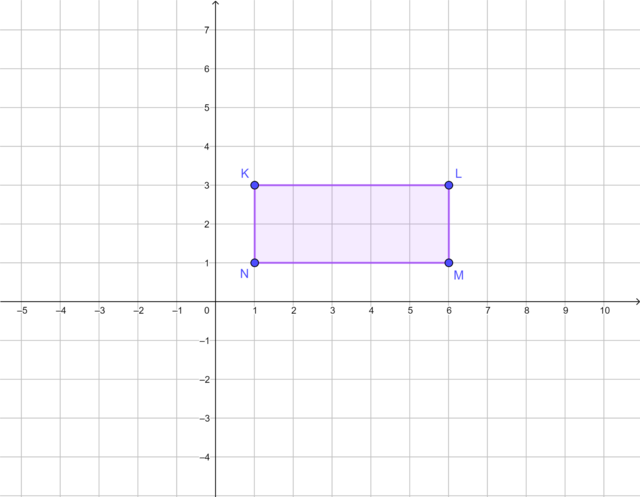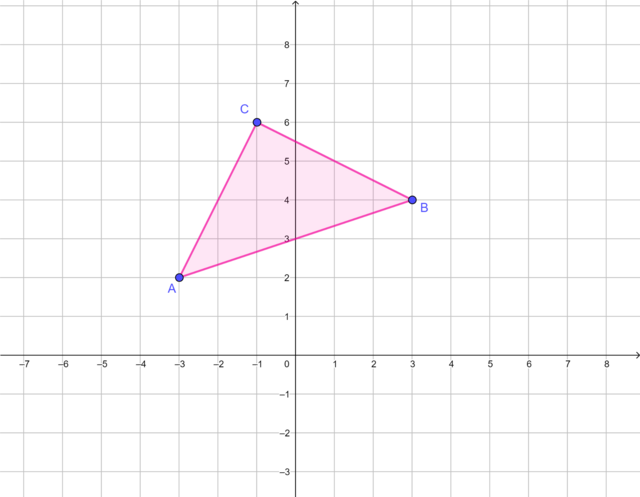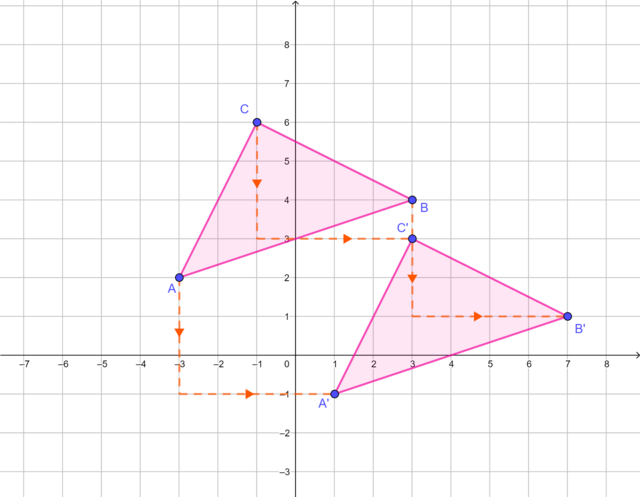Home Practice
For learners and parents For teachers and schools
Textbooks
Full catalogue
Pricing SupportLog in

We think you are located in United States. Is this correct?

# Translation transformations

## 24.4 Translation transformations

### Translating a point on the Cartesian plane

We can translate a point on the Cartesian plane from one position to another. For example, point $$A(1; 0)$$ is translated 2 units to the right and 4 units upwards.

The position of point $$A$$ after the transformation is called the image of $$A$$ and we use the notation $$A'$$ (we say "A prime").

image
the new position of a point, a line or an object after it has been transformed

$$A'$$ is at $$(3; 4)$$.

$$A'$$ is the image of $$A$$ under a translation transformation.

## Worked example 24.2: Translating a point on the Cartesian plane

Write down the coordinates of $$T'$$ if $$T(2; 4)$$ is translated $$3$$ units to the left and $$2$$ units downwards.

### Complete the horizontal translation.

We move point $$T$$ three units to the left (parallel to the $$x$$-axis). This translation moves $$T$$ from the first quadrant to the second quadrant.

### Complete the vertical translation.

We move point $$T$$ two units down (parallel to the $$y$$-axis).

The coordinates of $$T'$$ are $$(-1; 2)$$ and $$T'$$ lies in the second quadrant.

temp text

### Translating a shape on the Cartesian plane

We can translate a shape on the Cartesian plane from one position to another. For example:

• $$\triangle{ABC}$$ is translated 2 units to the left and 2 units downwards
• $$\triangle{A'B'C'}$$ is the image of $$\triangle{ABC}$$ under a translation transformation.Notice that each vertex of $$\triangle{ABC}$$ has been transformed by the same rule: slide 2 units to the left and 2 units downwards.

We can express this transformation rule as:

$(x; y) \rightarrow (x - 2; y - 2)$

This is called a mapping notation.

mapping notation
a representation of the rule that relates every point on the shape to the corresponding point on the image

### Finding the image of a rectangle under a translation transformation

The diagram shows rectangle $$KLMN$$ on the Cartesian plane.1. Draw a copy of rectangle $$KLMN$$ on a Cartesian plane.

2. Draw $$K'L'M'N'$$, the image of $$KLMN$$, translated 3 units to the right and 2 units upwards.

3. Express this translation transformation in mapping notation.

4. Compare the length of the corresponding sides of rectangle $$KLMN$$ and rectangle $$K'L'M'N'$$. What do you notice?

5. Compare the size of the corresponding interior angles of rectangle $$KLMN$$ and rectangle $$K'L'M'N'$$. What do you notice?

6. How would you describe these two shapes? Are they congruent shapes or similar shapes?

7. Calculate the perimeter of rectangle $$KLMN$$ and the perimeter of rectangle $$K'L'M'N'$$.

8. Calculate the area of rectangle $$KLMN$$ and the area of rectangle $$K'L'M'N'$$.

9. A translation transformation produces congruent shapes which have the same area and the same perimeter. Is this statement true or false?

## Worked example 24.3: Translating a triangle on the Cartesian plane

1. Draw $$\triangle ABC$$ with vertices $$A(-3; 2)$$, $$B(3; 4)$$ and $$C(-1; 6)$$ on the Cartesian plane.
2. Draw $$\triangle A'B'C'$$, the image of $$\triangle ABC$$, translated 4 units to the right and 3 units downwards.
3. Write down the coordinates of the vertices $$A'$$, $$B'$$ and $$C'$$.
4. Express this translation transformation in mapping notation.

### Draw the triangle on the Cartesian plane.### Translate each vertex of the triangle.

Translate each vertex of the $$\triangle ABC$$ 4 units to the right and 3 units downwards to get the image $$\triangle A'B'C'$$.### Write down the coordinates of the vertices of the image.

$A'(1; -1), B'(7; 1) \text{ and } C'(3; 3)$

### Write the transformation in mapping notation.

$(x; y) \rightarrow (x + 4; x - 3)$
temp text